# How to Calculate and Solve for Apparent Shear Stress | Slit Die | Polymer & Textile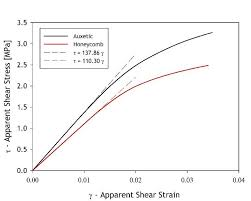The image above represents the apparent shear stress | slit die.

To compute for apparent shear stress | slit die, three essential parameters are needed and these parameters are height of die (h), pressure gradient (ΔP) and flow length (L).

The formula for calculating the apparent shear stress | slit die:

τapp = h . ΔP / 2L

Where:

τapp = Apparent Shear Stress | Slit Die
h = Height of Die
L = Flow Length

Let’s solve an example;
Find the apparent shear stress | slit die when the height of die is 7, pressure gradient is 12 and the flow length is 16.

This implies that;

h = Height of Die = 7
ΔP = Pressure Gradient = 12
L = Flow Length = 16

τapp = h . ΔP / 2L
τapp = 7 . (12) / 2(16)
τapp = 84 / 32
τapp = 2.625

Therefore, the apparent shear stress is 2.625.

Calculating the Height of Die when the Apparent Shear Stress, Pressure Gradient and the Flow Length is Given.

h = τapp x 2L / ΔP

Where:

h = Height of Die
τapp = Apparent Shear Stress | Slit Die
L = Flow Length

Let’s solve an example;
Find the height of die when the apparent shear stress | slit die is 32, the pressure gradient is 10 and the flow length is 8.

This implies that;

τapp = Apparent Shear Stress | Slit Die = 32
ΔP = Pressure Gradient = 10
L = Flow Length = 8

h = τapp x 2L / ΔP
h = 32 x 2 (8) / 10
h = 32 x 16 / 10
h = 512 / 10
h = 51.2

Therefore, the height of die is 51.2.

Calculating the Pressure Gradient when the Apparent Shear Stress, Height of Die and the Flow of Length is Given.

ΔP = τapp x 2L / h

Where:

τapp = Apparent Shear Stress | Slit Die
h = Height of Die
L = Flow Length

Let’s solve an example;
Find the pressure gradient when the apparent shear stress | slit die is 16, the height of die is 4 and the flow length is 2.

This implies that;

τapp = Apparent Shear Stress | Slit Die = 16
h = Height of Die = 4
L = Flow Length = 2

ΔP = τapp x 2L / h
ΔP = 16 x 2 (2) / 4
ΔP = 16 x 4 / 4
ΔP = 64 / 4
ΔP = 16

Therefore, the pressure gradient is 16.

Calculating the Flow Length when the Apparent Shear Stress when the Height of Die and the Pressure Gradient is Given.

L = h . ΔP / τapp x 2

Where:

L = Flow Length
τapp = Apparent Shear Stress | Slit Die
h = Height of Die

Let’s solve an example;
Find the flow length when the apparent shear stress | slit die is 15, the height of die is 10 and the pressure gradient is 4.

This implies that;

τapp = Apparent Shear Stress | Slit Die = 15
h = Height of Die = 10
ΔP = Pressure Gradient = 4

L = h . ΔP / τapp x 2
L = 10 . 4 / 15 x 2
L = 40 / 30
L = 1.33

Therefore, the flow length is 1.33.

Nickzom Calculator – The Calculator Encyclopedia is capable of calculating the apparent shear stress | slit die.

To get the answer and workings of the apparent shear stress | slit die using the Nickzom Calculator – The Calculator Encyclopedia. First, you need to obtain the app.

You can get this app via any of these means:

To get access to the professional version via web, you need to register and subscribe for NGN 1,500 per annum to have utter access to all functionalities.
You can also try the demo version via https://www.nickzom.org/calculator

Apple (Paid) – https://itunes.apple.com/us/app/nickzom-calculator/id1331162702?mt=8
Once, you have obtained the calculator encyclopedia app, proceed to the Calculator Map, then click on Polymer & Textile under Engineering.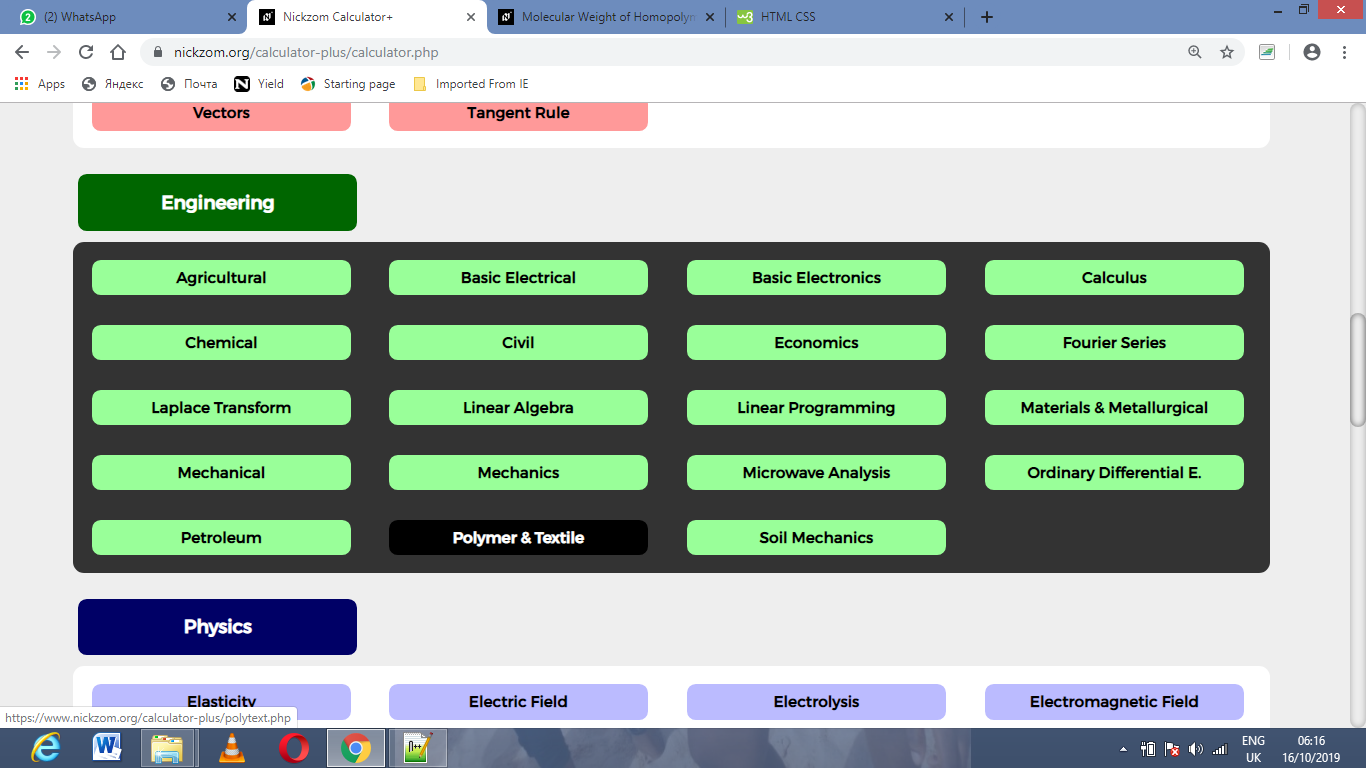Now, Click on Apparent Shear Stress| Slit Die under Polymer & Textile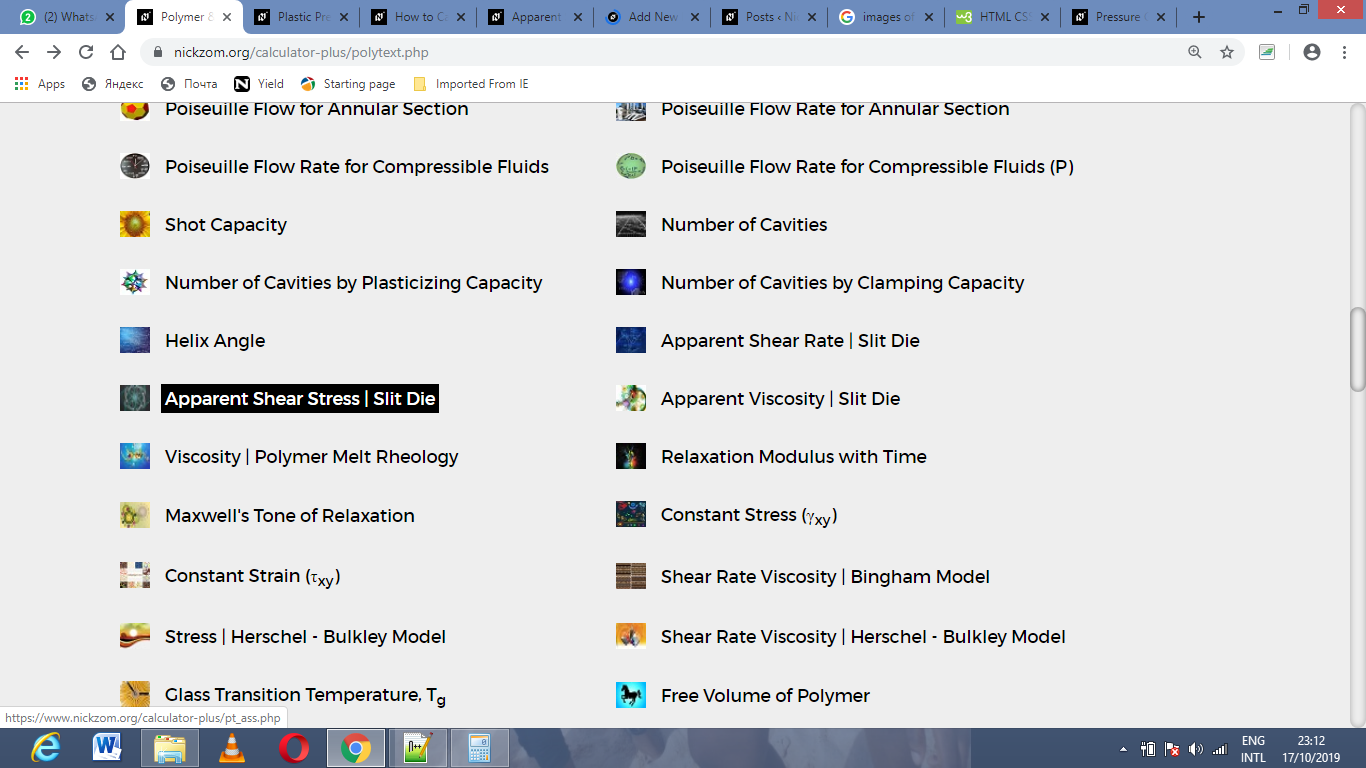The screenshot below displays the page or activity to enter your values, to get the answer for the apparent shear stress | slit die according to the respective parameters which is the height of die (h), pressure gradient (ΔP) and flow length (L).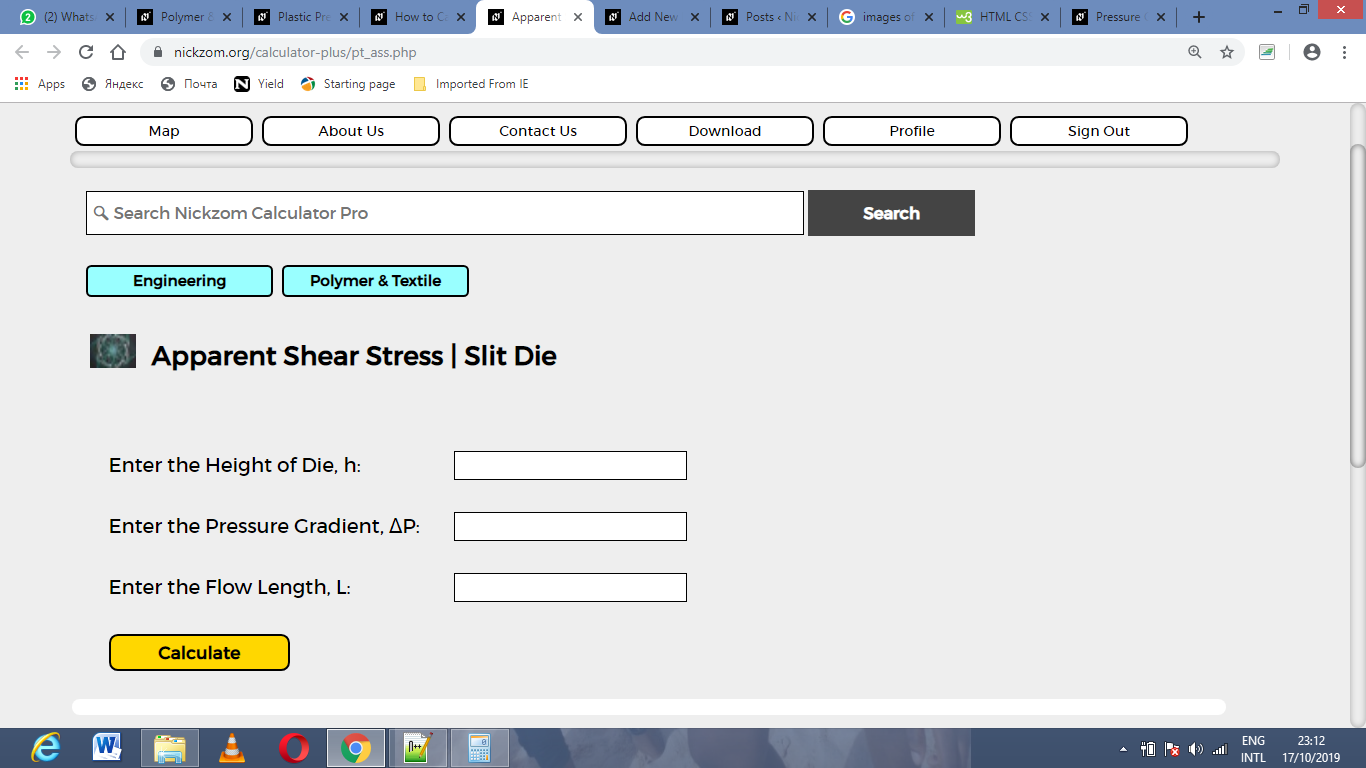Now, enter the values appropriately and accordingly for the parameters as required by the height of die (h) is 7, pressure gradient (ΔP) is 12 and flow length (L) is 16.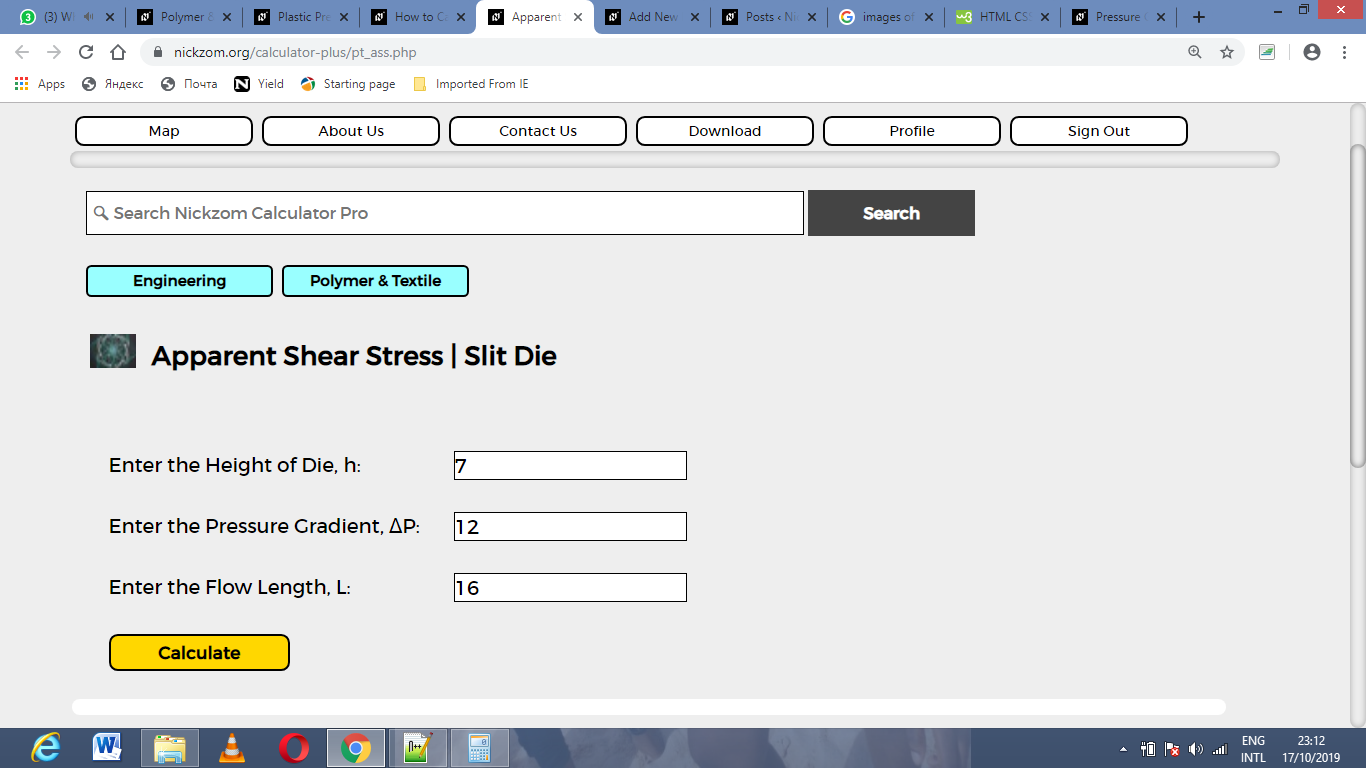Finally, Click on Calculate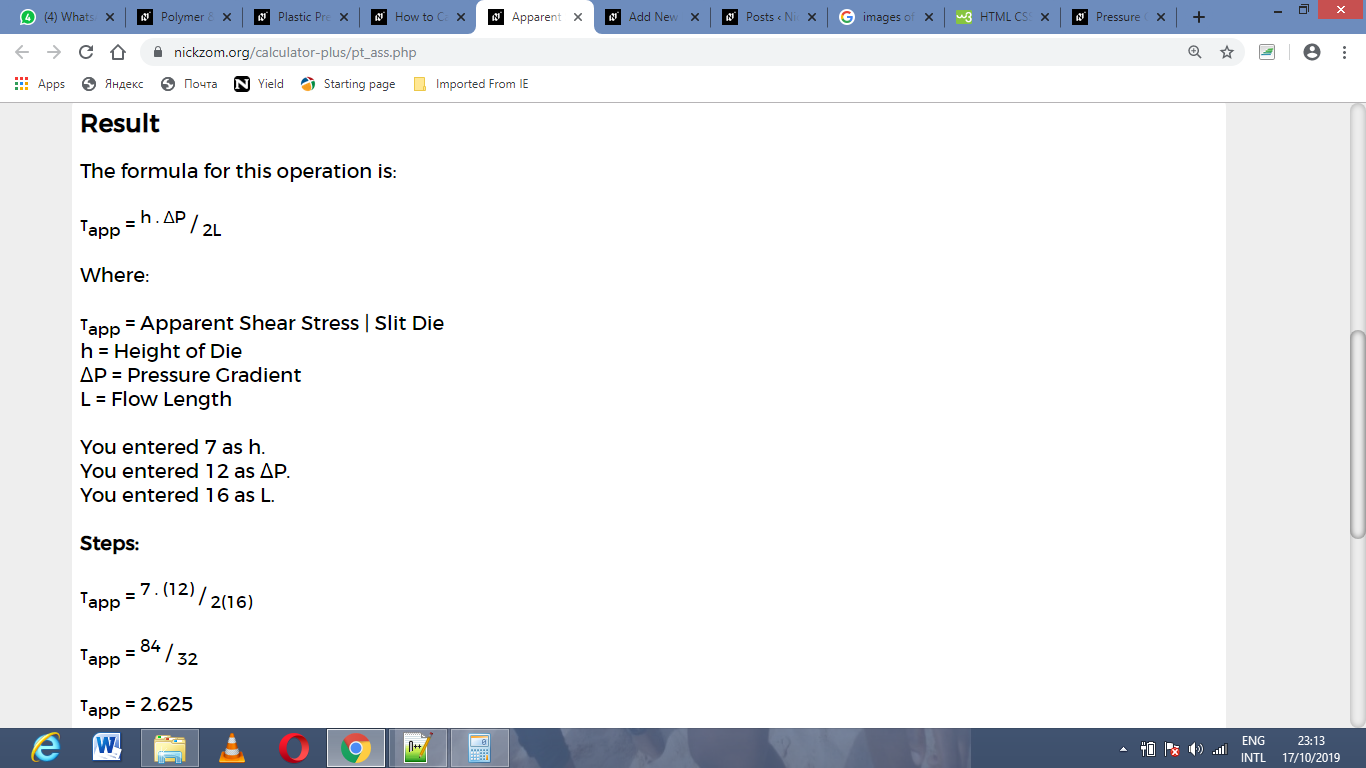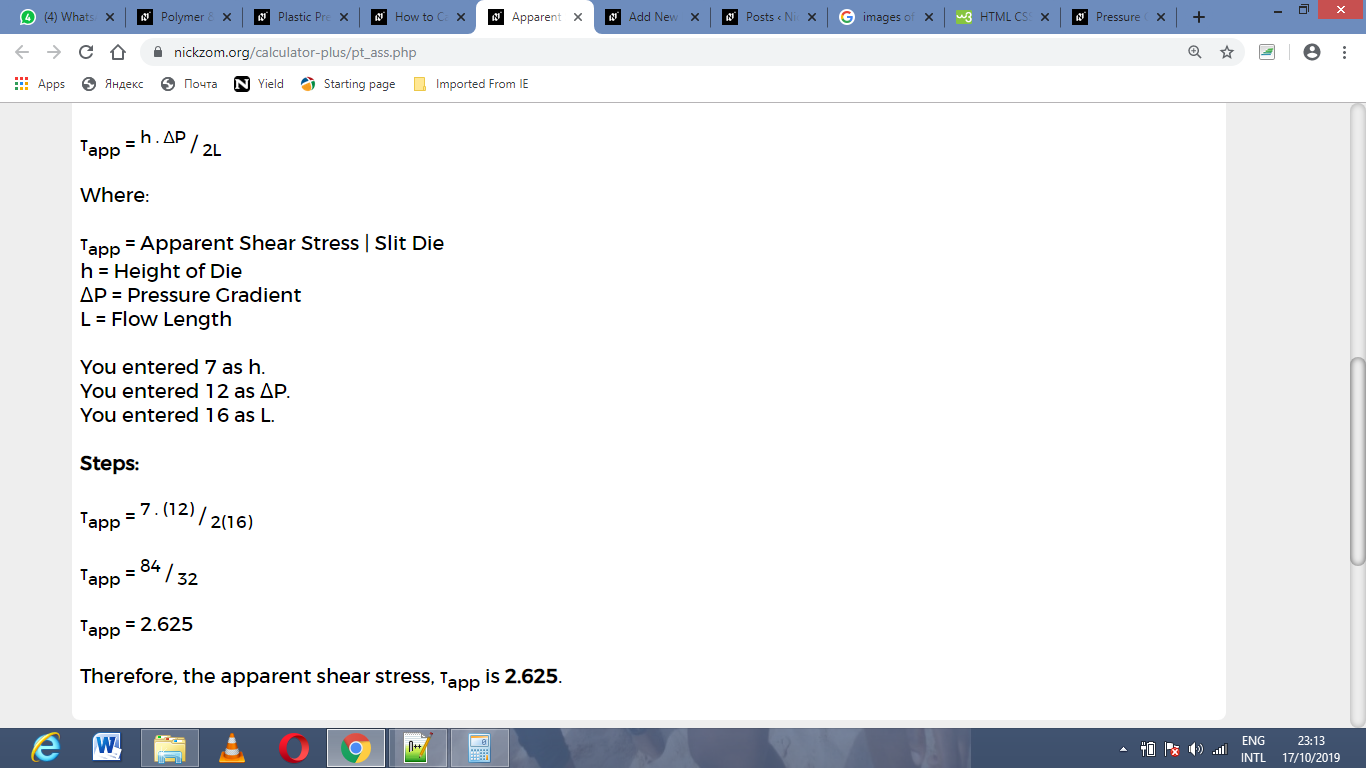As you can see from the screenshot above, Nickzom Calculator– The Calculator Encyclopedia solves for the apparent shear stress | slit die and presents the formula, workings and steps too.